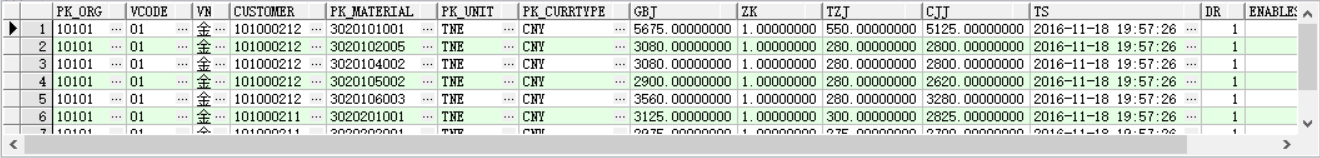`````` create or replace view imp_stockprice as
select c.code as pk_org,b.vcode,b.vname,d.code as customer,e.code as pk_material,f.code as pk_unit,g.code as pk_currtype,
a.nprice1 as gbj,a.nprice2 as zk,a.nprice3 as tzj,a.nprice4 as cjj,substr(a.ts,0,20) as ts,a.dr,e.enablestate
from prm_tariff a
left join prm_tariffdef b on b.pk_tariffdef=a.pk_tariffdef
left join org_orgs c on c.pk_org=a.pk_org
left join bd_customer d on d.pk_customer=a.pk_customer
left join bd_material e on e.pk_material=a.pk_material
left join bd_measdoc f on f.pk_measdoc=a.pk_unit
left join bd_currtype g on g.pk_currtype=a.pk_currtype
where e.setpartsflag='N'and b.dr=0 and d.enablestate=2 and d.dr=0 and c.enablestate=2;
``````2个回答